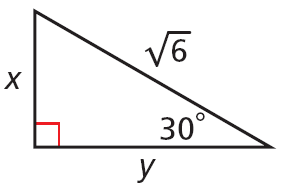# Right Triangles and Trigonometry

## Objective

Multiply and divide radicals. Rationalize the denominator.

## Common Core Standards

### Core Standards

?

• A.SSE.A.2 — Use the structure of an expression to identify ways to rewrite it. For example, see x4 — y4 as (x²)² — (y²)², thus recognizing it as a difference of squares that can be factored as (x² — y²)(x² + y²).

• N.RN.A.2 — Rewrite expressions involving radicals and rational exponents using the properties of exponents.

?

• 8.EE.A.2

## Criteria for Success

?

1. Multiply and divide radicals by following properties of radicals. For example: ${3\sqrt{7}\cdot2\sqrt{5}=\left(2\cdot3\sqrt{(7\cdot5)}\right)}$
2. Describe that radicals follow the same rules as exponents with power of a power and power of a quotient. For example: ${\sqrt{\left(\frac{2}{3}\right)}=\frac{\sqrt{2}}{\sqrt{3}}}$
3. Rationalize the denominator in a radical expression when there is a radical term in the denominator in algebraic expressions.
4. Identify when it is proper to "rationalize the denominator."
5. Rationalize the denominator in a radical expression when there is a radical term in the denominator in geometric problems with special right triangles.
6. Calculate the area of right triangles.

## Tips for Teachers

?

• This lesson extends work done in Algebra 1. The properties of radicals should be familiar to students but will need some review.
• The focus of this lesson is on working with numeric radical expressions, but students should practice with algebraic radical expressions as well.

## Anchor Problems

?

### Problem 1

Simplify the following:

${\sqrt{32}}$

${\sqrt{5^{10}}}$

${\sqrt{4x^4}}$

#### References

EngageNY Mathematics Geometry > Module 2 > Topic D > Lesson 22Exercise 2 and 3

Geometry > Module 2 > Topic D > Lesson 22 of the New York State Common Core Mathematics Curriculum from EngageNY and Great Minds. © 2015 Great Minds. Licensed by EngageNY of the New York State Education Department under the CC BY-NC-SA 3.0 US license. Accessed Dec. 2, 2016, 5:15 p.m..

Modified by Fishtank Learning, Inc.

### Problem 2

Each of these statements are TRUE for some values. Identify the excluded values, then describe what the statement says about the property. Feel free to use an example.

Statement 1:   ${\sqrt{ab}=\sqrt{a}\cdot\sqrt{b}}$

Statement 2:   ${\sqrt{\frac{a}{b}}=\frac{\sqrt{a}}{\sqrt{b}}=\frac{\sqrt{ab}}{b}}$

Statement 3:   ${c\sqrt{a}\cdot d\sqrt{b}=cd\sqrt{ab}}$

### Problem 3

Find the area of the triangle below.## Problem Set

?

The following resources include problems and activities aligned to the objective of the lesson that can be used to create your own problem set.

• Include problems where students need to identify the form of expression that is most useful given the goal of the problem.
• Include error analysis problems, such as “What’s the mistake? ${\frac{\sqrt{2a}}{2}=a}$” or with a specific example “What is the mistake? ${\frac{\sqrt{6}}{2}=\sqrt{3}}$.”
• Include problems where there are variable expressions in the radicand.
• Include problems where students need to find a missing measurement of a right triangle, including using special right triangles.
• Include problems where one of the sides of a right triangle is given in radical form and students need to find the area of the triangle, including using special right triangles, similar to Anchor Problem #3.

?

### Problem 1

What is the value of $x$ that will make the following equation true? Explain your reasoning.

$\sqrt{5}\cdot3\sqrt{x}=15\sqrt{6}$

### Problem 2

Write each expression in its simplest radical form.

${\sqrt{243}=}$

${\sqrt\frac{7}{5}=}$

#### References

EngageNY Mathematics Geometry > Module 2 > Topic D > Lesson 22Exit Ticket, Question #1 and #2

Geometry > Module 2 > Topic D > Lesson 22 of the New York State Common Core Mathematics Curriculum from EngageNY and Great Minds. © 2015 Great Minds. Licensed by EngageNY of the New York State Education Department under the CC BY-NC-SA 3.0 US license. Accessed Dec. 2, 2016, 5:15 p.m..